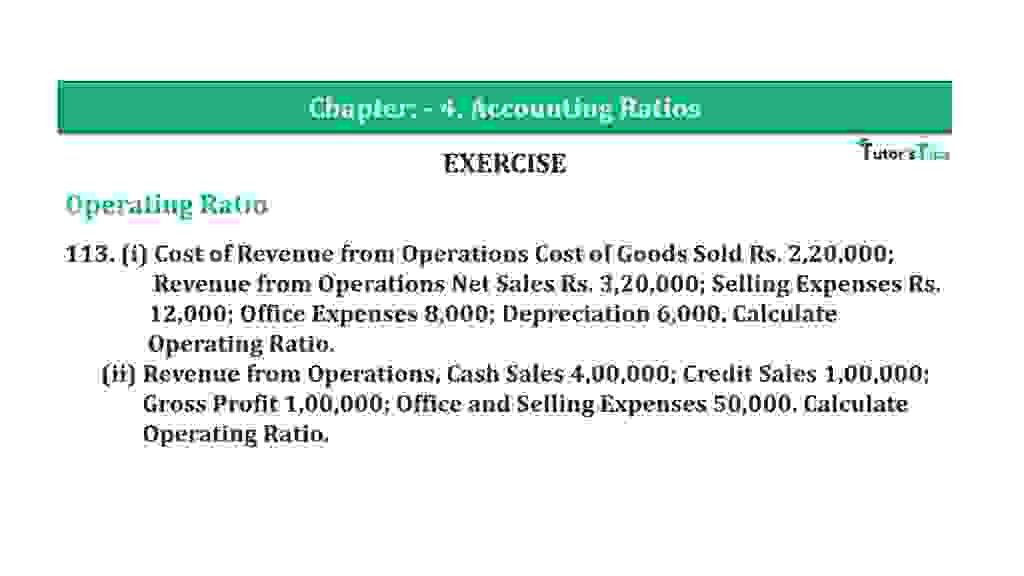# Question 113 Chapter 4 of +2-B – T.S. Grewal 12 ClassQuestion 113 Chapter 4 of +2-B

Operating Ratio

113. (i) Cost of Revenue from Operations Cost of Goods Sold Rs. 2,20,000;
Revenue from Operations Net Sales Rs. 3,20,000; Selling Expenses Rs.
12,000; Office Expenses 8,000; Depreciation 6,000. Calculate
Operating Ratio.
(ii) Revenue from Operations, Cash Sales 4,00,000; Credit Sales 1,00,000;
Gross Profit 1,00,000; Office and Selling Expenses 50,000. Calculate
Operating Ratio.

### The solution of Question 113 Chapter 4 of +2-B: –

Case I :

 Net Sales = Rs. 3,20,000 Operating Expenses = Selling Expenses + Office Expenses + Depreciation = Rs. 12,000 + Rs. 8,000 + Rs. 6,000 = Rs. 26,000 Cost of Goods Sold = Rs. 2,20,000 Operating Cost = Cost of Goods Sold + Operating Expenses = Rs. 2,20,000 + Rs. 26,000 = Rs. 2,46,000

 Operating Ratio = Operating Cost X 100 Net Sales
 = 2,46,000 X 100 Rs. 3,20,000 = 76.87%

Case II:

 Net Sales = Cash Sales + Credit Sales = Rs. 4,00,000 + Rs. 1,00,000 = Rs. 5,00,000 Cost of Goods Sold = Net Sales – Gross Profit = Rs. 5,00,000 + Rs. 1,00,000 = Rs. 4,00,000 Operating Expenses = Rs. 50,000 Operating Cost = Cost of Goods Sold + Operating Expenses = Rs. 4,00,000 + Rs. 50,000 = Rs. 4,50,000

 Operating Ratio = Operating Cost X 100 Net Sales
 = Rs. 4,50,000 X 100 Rs. 5,00,000 = 90%

Balance Sheet: Meaning, Format & Examples

Thanks, Please Like and share with your friends

Comment if you have any question.

Also, Check out the solved question of previous Chapters: –

### T.S. Grewal’s Double Entry Book Keeping (Vol. II: Accounting for Companies)T.S. Grewal’s Analysis of Financial Statements